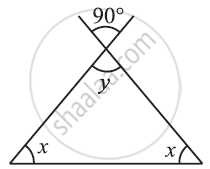Share

# Find the Value of the Unknowns X And Y In the Following Diagram: - 5 - CBSE Class 7 - Mathematics

ConceptAngle Sum Property of a Triangle

#### Question

Find the value of the unknowns x and y in the following diagram:#### Solution

y = 90° (Vertically opposite angles)

y = 180° (Angle sum property)

2x + y = 180°

2x + 90° = 180°

2x = 180° − 90° = 90°

x = 90^@/2 = 45^@

Is there an error in this question or solution?

#### APPEARS IN

NCERT Solution for Mathematics for Class 7 (2018 to Current)
Chapter 6: The Triangle and its Properties
Ex. 6.30 | Q: 2.5 | Page no. 121
Solution Find the Value of the Unknowns X And Y In the Following Diagram: - 5 Concept: Angle Sum Property of a Triangle.
S